Next: Nonlinear factor analysis and Up: Missing Values in Hierarchical Previous: Introduction

# Variational Bayesian learning for nonlinear models

Variational Bayesian (VB) learning techniques are based on approximating the true posterior probability density of the unknown variables of the model by a function with a restricted form. Currently the most common technique is ensemble learning  where Kullback-Leibler divergence measures the misfit between the approximation and the true posterior. It has been applied to ICA and a wide variety of other models (see [1,9] for some references).

In ensemble learning, the posterior approximation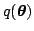of the unknown variables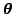is required to have a suitably factorial form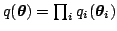, whereare the subsets of unknown variables. The misfit between the true posterior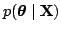and its approximationis measured by Kullback-Leibler divergence. An additional term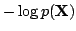is included to avoid calculation of the model evidence term. The cost function is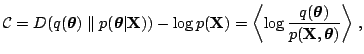(2)

wheredenotes the expectation over distribution. Note that since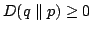, it follows that the cost function provides a lower bound for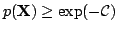. For a more detailed discussion, see .

The missing values in data behave like other latent variables and are therefore handled as a part ofinstead of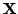. The posterior approximationis estimated during the learning and it can be used as a reconstruction for the missing values. The fraction of missing values in the data does not affect computational complexity substantially.

Beal and Ghahramani  compare the VB method of handling incomplete data to annealed importance sampling (AIS). In their example, the variational method works more reliably and about 100 times faster than AIS. Chan et al.  used ICA with VB learning successfully to reconstruct missing values. A competing approach without VB by Welling and Weber  has an exponential complexity w.r.t. the data dimensionality. ICA can be seen as FA with a non-Gaussian source model. Instead of going into that direction, we choose to stick to the Gaussian source model and concentrate on extending the mapping to be nonlinear instead.

SubsectionsNext: Nonlinear factor analysis and Up: Missing Values in Hierarchical Previous: Introduction
Tapani Raiko 2003-07-01